Publicité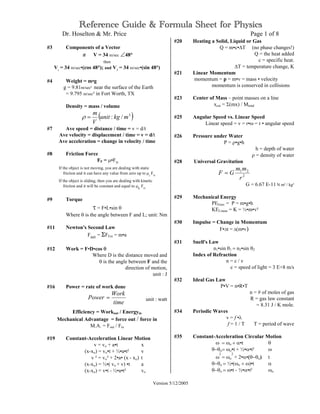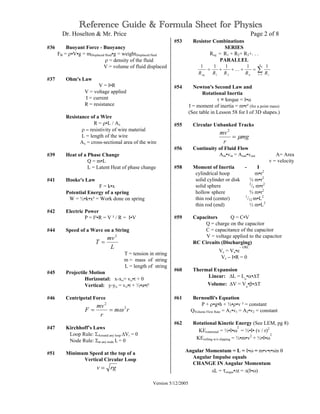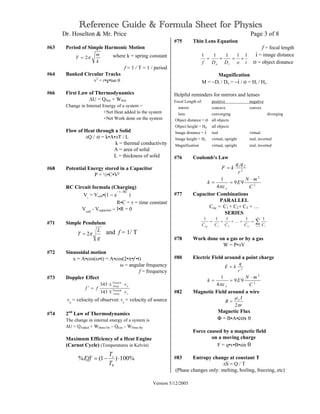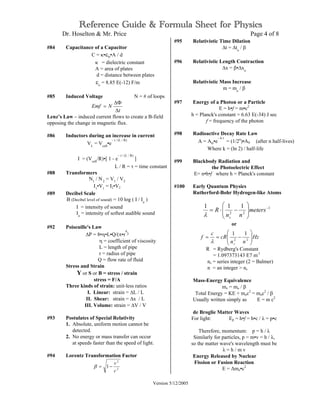Publicité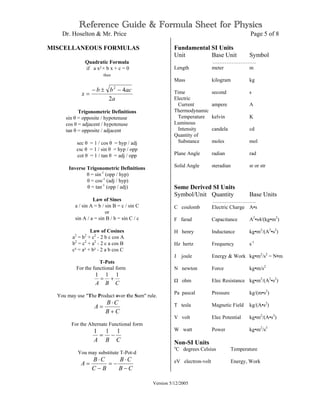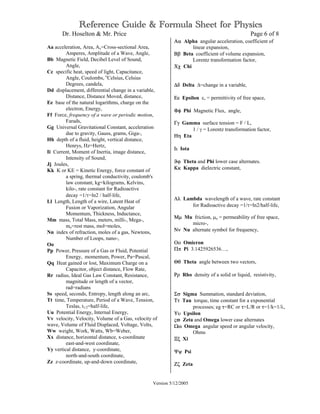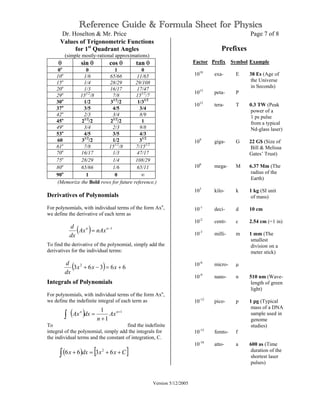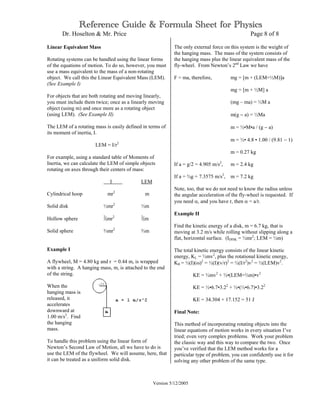Prochain SlideShare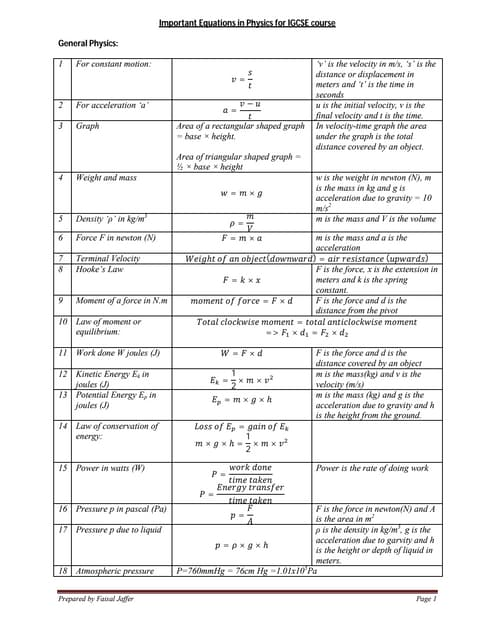Important equation in physics2
Chargement dans ... 3
1 sur 8
Publicité

### Physics Formula list (1)

1. Reference Guide & Formula Sheet for Physics Dr. Hoselton & Mr. Price Page 1 of 8 Version 5/12/2005 #3 Components of a Vector if V = 34 m/sec ∠48° then Vi = 34 m/sec•(cos 48°); and VJ = 34 m/sec•(sin 48°) #4 Weight = m•g g = 9.81m/sec² near the surface of the Earth = 9.795 m/sec² in Fort Worth, TX Density = mass / volume ( )3 /: mkgunit V m =ρ #7 Ave speed = distance / time = v = d/t Ave velocity = displacement / time = v = d/t Ave acceleration = change in velocity / time #8 Friction Force FF = µ•FN If the object is not moving, you are dealing with static friction and it can have any value from zero up to µs FN If the object is sliding, then you are dealing with kinetic friction and it will be constant and equal to µK FN #9 Torque τ = F•L•sin θ Where θ is the angle between F and L; unit: Nm #11 Newton's Second Law Fnet = ΣFExt = m•a #12 Work = F•D•cos θ Where D is the distance moved and θ is the angle between F and the direction of motion, unit : J #16 Power = rate of work done unit : watt Efficiency = Workout / Energyin Mechanical Advantage = force out / force in M.A. = Fout / Fin #19 Constant-Acceleration Linear Motion v = vο + a•t x (x-xο) = vο•t + ½•a•t² v v ² = vο² + 2•a• (x - xο) t (x-xο) = ½•( vο + v) •t a (x-xο) = v•t - ½•a•t² vο #20 Heating a Solid, Liquid or Gas Q = m•c•∆T (no phase changes!) Q = the heat added c = specific heat. ∆T = temperature change, K #21 Linear Momentum momentum = p = m•v = mass • velocity momentum is conserved in collisions #23 Center of Mass – point masses on a line xcm = Σ(mx) / Mtotal #25 Angular Speed vs. Linear Speed Linear speed = v = r•ω = r • angular speed #26 Pressure under Water P = ρ•g•h h = depth of water ρ = density of water #28 Universal Gravitation 2 21 r mm GF = G = 6.67 E-11 N m² / kg² #29 Mechanical Energy PEGrav = P = m•g•h KELinear = K = ½•m•v² #30 Impulse = Change in Momentum F•∆t = ∆(m•v) #31 Snell's Law n1•sin θ1 = n2•sin θ2 Index of Refraction n = c / v c = speed of light = 3 E+8 m/s #32 Ideal Gas Law P•V = n•R•T n = # of moles of gas R = gas law constant = 8.31 J / K mole. #34 Periodic Waves v = f •λ f = 1 / T T = period of wave #35 Constant-Acceleration Circular Motion ω = ωο + α•t θ θ−θο= ωο•t + ½•α•t² ω ω 2 = ωο 2 + 2•α•(θ−θο) t θ−θο = ½•(ωο + ω)•t α θ−θο = ω•t - ½•α•t² ωο time Work Power =
2. Reference Guide & Formula Sheet for Physics Dr. Hoselton & Mr. Price Page 2 of 8 Version 5/12/2005 #36 Buoyant Force - Buoyancy FB = ρ•V•g = mDisplaced fluid•g = weightDisplaced fluid ρ = density of the fluid V = volume of fluid displaced #37 Ohm's Law V = I•R V = voltage applied I = current R = resistance Resistance of a Wire R = ρ•L / Ax ρ = resistivity of wire material L = length of the wire Ax = cross-sectional area of the wire #39 Heat of a Phase Change Q = m•L L = Latent Heat of phase change #41 Hooke's Law F = k•x Potential Energy of a spring W = ½•k•x² = Work done on spring #42 Electric Power P = I²•R = V ² / R = I•V #44 Speed of a Wave on a String L mv T 2 = T = tension in string m = mass of string L = length of string #45 Projectile Motion Horizontal: x-xο= vο•t + 0 Vertical: y-yο = vο•t + ½•a•t² #46 Centripetal Force rm r mv F 2 2 ω== #47 Kirchhoff’s Laws Loop Rule: ΣAround any loop ∆Vi = 0 Node Rule: Σat any node Ii = 0 #51 Minimum Speed at the top of a Vertical Circular Loop rgv = #53 Resistor Combinations SERIES Req = R1 + R2+ R3+. . . PARALLEL ∑= =+++= n i ineq RRRRR 121 11111 K #54 Newton's Second Law and Rotational Inertia τ = torque = I•α I = moment of inertia = m•r² (for a point mass) (See table in Lesson 58 for I of 3D shapes.) #55 Circular Unbanked Tracks mg r mv µ= 2 #56 Continuity of Fluid Flow Ain•vin = Aout•vout A= Area v = velocity #58 Moment of Inertia - I cylindrical hoop m•r2 solid cylinder or disk ½ m•r2 solid sphere 2 /5 m•r2 hollow sphere ⅔ m•r2 thin rod (center) 1 /12 m•L2 thin rod (end) ⅓ m•L2 #59 Capacitors Q = C•V Q = charge on the capacitor C = capacitance of the capacitor V = voltage applied to the capacitor RC Circuits (Discharging) Vc = Vo•e − t/RC Vc − I•R = 0 #60 Thermal Expansion Linear: ∆L = Lo •α•∆T Volume: ∆V = Vo •β•∆T #61 Bernoulli's Equation P + ρ•g•h + ½•ρ•v ² = constant QVolume Flow Rate = A1•v1 = A2•v2 = constant #62 Rotational Kinetic Energy (See LEM, pg 8) KErotational = ½•I•ω 2 = ½•I• (v / r)2 KErolling w/o slipping = ½•m•v2 + ½•I•ω 2 Angular Momentum = L = I•ω = m•v•r•sin θ Angular Impulse equals CHANGE IN Angular Momentum ∆L = τorque•∆t = ∆(I•ω)
3. Reference Guide & Formula Sheet for Physics Dr. Hoselton & Mr. Price Page 3 of 8 Version 5/12/2005 #63 Period of Simple Harmonic Motion k m T π2= where k = spring constant f = 1 / T = 1 / period #64 Banked Circular Tracks v2 = r•g•tan θ #66 First Law of Thermodynamics ∆U = QNet + WNet Change in Internal Energy of a system = +Net Heat added to the system +Net Work done on the system Flow of Heat through a Solid ∆Q / ∆t = k•A•∆T / L k = thermal conductivity A = area of solid L = thickness of solid #68 Potential Energy stored in a Capacitor P = ½•C•V² RC Circuit formula (Charging) Vc = Vcell•(1 − e − t / RC ) R•C = τ = time constant Vcell - Vcapacitor − I•R = 0 #71 Simple Pendulum g L T π2= and f = 1/ T #72 Sinusoidal motion x = A•cos(ω•t) = A•cos(2•π•f •t) ω = angular frequency f = frequency #73 Doppler Effect s Toward Away o Toward Away v v ff m343 343 ± =′ vo = velocity of observer: vs = velocity of source #74 2nd Law of Thermodynamics The change in internal energy of a system is ∆U = QAdded + WDone On – Qlost – WDone By Maximum Efficiency of a Heat Engine (Carnot Cycle) (Temperatures in Kelvin) #75 Thin Lens Equation f = focal length i = image distance o = object distance Magnification M = −Di / Do = −i / o = Hi / Ho Helpful reminders for mirrors and lenses Focal Length of: positive negative mirror concave convex lens converging diverging Object distance = o all objects Object height = Ho all objects Image distance = i real virtual Image height = Hi virtual, upright real, inverted Magnification virtual, upright real, inverted #76 Coulomb's Law 2 21 r qq kF = 2 2 99 4 1 C mN Ek o ⋅ == πε #77 Capacitor Combinations PARALLEL Ceq = C1 + C2+ C3 + … SERIES ∑= =+++= n i ineq CCCCC 121 11111 K #78 Work done on a gas or by a gas W = P•∆V #80 Electric Field around a point charge 2 r q kE = 2 2 99 4 1 C mN Ek o ⋅ == πε #82 Magnetic Field around a wire r I B o π µ 2 = Magnetic Flux Φ = B•A•cos θ Force caused by a magnetic field on a moving charge F = q•v•B•sin θ #83 Entropy change at constant T ∆S = Q / T (Phase changes only: melting, boiling, freezing, etc) %100)1(% ⋅−= h c T T Eff ioDDf io 11111 +=+=
4. Reference Guide & Formula Sheet for Physics Dr. Hoselton & Mr. Price Page 4 of 8 Version 5/12/2005 #84 Capacitance of a Capacitor C = κ•εo•A / d κ = dielectric constant A = area of plates d = distance between plates εo = 8.85 E(-12) F/m #85 Induced Voltage N = # of loops t NEmf ∆ ∆Φ = Lenz’s Law – induced current flows to create a B-field opposing the change in magnetic flux. #86 Inductors during an increase in current VL = Vcell •e − t / (L / R) I = (Vcell /R)•[ 1 - e − t / (L / R) ] L / R = τ = time constant #88 Transformers N1 / N2 = V1 / V2 I1 •V1 = I2•V2 #89 Decibel Scale B (Decibel level of sound) = 10 log ( I / Io ) I = intensity of sound Io = intensity of softest audible sound #92 Poiseuille's Law ∆P = 8•η•L•Q/(π•r 4 ) η = coefficient of viscosity L = length of pipe r = radius of pipe Q = flow rate of fluid Stress and Strain Y or S or B = stress / strain stress = F/A Three kinds of strain: unit-less ratios I. Linear: strain = ∆L / L II. Shear: strain = ∆x / L III. Volume: strain = ∆V / V #93 Postulates of Special Relativity 1. Absolute, uniform motion cannot be detected. 2. No energy or mass transfer can occur at speeds faster than the speed of light. #94 Lorentz Transformation Factor 2 2 1 c v −=β #95 Relativistic Time Dilation ∆t = ∆to / β #96 Relativistic Length Contraction ∆x = β•∆xo Relativistic Mass Increase m = mo / β #97 Energy of a Photon or a Particle E = h•f = m•c2 h = Planck's constant = 6.63 E(-34) J sec f = frequency of the photon #98 Radioactive Decay Rate Law A = Ao•e − k t = (1/2n )•A0 (after n half-lives) Where k = (ln 2) / half-life #99 Blackbody Radiation and the Photoelectric Effect E= n•h•f where h = Planck's constant #100 Early Quantum Physics Rutherford-Bohr Hydrogen-like Atoms or R = Rydberg's Constant = 1.097373143 E7 m-1 ns = series integer (2 = Balmer) n = an integer > ns Mass-Energy Equivalence mv = mo / β Total Energy = KE + moc2 = moc2 / β Usually written simply as E = m c2 de Broglie Matter Waves For light: Ep = h•f = h•c / λ = p•c Therefore, momentum: p = h / λ Similarly for particles, p = m•v = h / λ, so the matter wave's wavelength must be λ = h / m v Energy Released by Nuclear Fission or Fusion Reaction E = ∆mo•c2 Hz nn cR c f s         −== 22 11 λ 1 22 111 −         −⋅= meters nn R sλ